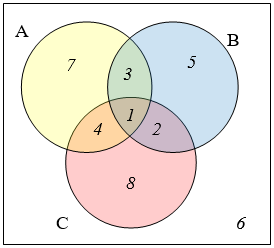$$\newcommand{\id}{\mathrm{id}}$$ $$\newcommand{\Span}{\mathrm{span}}$$ $$\newcommand{\kernel}{\mathrm{null}\,}$$ $$\newcommand{\range}{\mathrm{range}\,}$$ $$\newcommand{\RealPart}{\mathrm{Re}}$$ $$\newcommand{\ImaginaryPart}{\mathrm{Im}}$$ $$\newcommand{\Argument}{\mathrm{Arg}}$$ $$\newcommand{\norm}{\| #1 \|}$$ $$\newcommand{\inner}{\langle #1, #2 \rangle}$$ $$\newcommand{\Span}{\mathrm{span}}$$

# 13.5: Exercises

$$\newcommand{\vecs}{\overset { \rightharpoonup} {\mathbf{#1}} }$$ $$\newcommand{\vecd}{\overset{-\!-\!\rightharpoonup}{\vphantom{a}\smash {#1}}}$$$$\newcommand{\id}{\mathrm{id}}$$ $$\newcommand{\Span}{\mathrm{span}}$$ $$\newcommand{\kernel}{\mathrm{null}\,}$$ $$\newcommand{\range}{\mathrm{range}\,}$$ $$\newcommand{\RealPart}{\mathrm{Re}}$$ $$\newcommand{\ImaginaryPart}{\mathrm{Im}}$$ $$\newcommand{\Argument}{\mathrm{Arg}}$$ $$\newcommand{\norm}{\| #1 \|}$$ $$\newcommand{\inner}{\langle #1, #2 \rangle}$$ $$\newcommand{\Span}{\mathrm{span}}$$ $$\newcommand{\id}{\mathrm{id}}$$ $$\newcommand{\Span}{\mathrm{span}}$$ $$\newcommand{\kernel}{\mathrm{null}\,}$$ $$\newcommand{\range}{\mathrm{range}\,}$$ $$\newcommand{\RealPart}{\mathrm{Re}}$$ $$\newcommand{\ImaginaryPart}{\mathrm{Im}}$$ $$\newcommand{\Argument}{\mathrm{Arg}}$$ $$\newcommand{\norm}{\| #1 \|}$$ $$\newcommand{\inner}{\langle #1, #2 \rangle}$$ $$\newcommand{\Span}{\mathrm{span}}$$

1. List out the elements of the set “The letters of the word Mississipi”

2. List out the elements of the set “Months of the year”

3. Write a verbal description of the set $$\{3,6,9\}$$

4. Write a verbal description of the set $$\{a, i, e, 0, u\}$$

5. Is $$\{1,3,5\}$$ a subset of the set of odd integers?

6. Is $$\{A, B, C\}$$a subset of the set of letters of the alphabet?

For problems 7-12, consider the sets below, and indicate if each statement is true or false.

$$A=\{1,2,3,4,5\} \quad B=\{1,3,5\} \quad C=\{4,6\} \quad U=\{\text { numbers from } 0 \text { to } 10\}$$

7. $$3 \in B$$

8. $$5 \in C$$

9. $$B \subset A$$

10. $$C \subset A$$

11. $$C \subset B$$

12. $$C \subset U$$

Using the sets from above, and treating $$U$$ as the Universal set, find each of the following:

13. $$A \cup B$$

14. $$A \cup C$$

15. $$A \cap C$$

16. $$B \cap C$$

17. $$A^{c}$$

18. $$B^{c}$$

Let $$D=\{b, a, c, k\}, \quad E=\{t, a, s, k\}, \quad F=\{b, a, t, h\}$$. Using these sets, find the following:

19. $$D^{c} \cap E$$

20. $$F^{c} \cap D$$

21. $$(D \cap E) \cup F$$

22. $$D \cap(E \cup P)$$

23. $$(F \cap E)^{c} \cap D$$

24. $$(D \cup E)^{c} \cap F$$

Create a Venn diagram to illustrate each of the following:

25. $$(F \cap E) \cup D$$

26. $$(D \cup E)^{c} \cap F$$

27. $$\left(F^{c} \cap E^{\prime}\right) \cap D$$

28. $$(D \cup E) \cup F$$

Write an expression for the shaded region.

29.30.31.32.Let $$A=\{1,2,3,4,5\} \quad B=\{1,3,5\} \quad C=\{4,6\}$$. Find the cardinality of the given set.

33. $$\mathrm{n}(A)$$

34. $$\mathrm{n}(B)$$

35. $$\mathrm{n}(A \cup C)$$

36. $$\mathrm{n}(A \cap C)$$

The Venn diagram here shows the cardinality of each set. Use this in 37-40 to find the cardinality of given set.37. $$\mathrm{n}(A \cap C)$$

38. $$\mathrm{n}(B \cup C)$$

39. $$\mathrm{n}\left(A \cap B \cap C^{2}\right)$$

40. $$\mathrm{n}\left(A \cap B^{c} \cap C\right)$$

41. If $$n(G)=20, n(H)=30, n(G \cap H)=5,$$ find $$n(G \cup H)$$

42. If $$n(G)=5, n(H)=8, n(G \cap H)=4,$$ find $$n(G \cup H)$$

43. A survey was given asking whether they watch movies at home from Netflix, Redbox, or a video store. Use the results to determine how many people use Redbox.

$$\begin{array}{ll} \text{52 only use Netflix} & \text{62 only use Redbox} \\ \text{24 only use a video store} & \text{16 use only a video store and Redbox} \\ \text{48 use only Netflix and Redbox} & \text{30 use only a video store and Netflix} \\ \text{10 use all three} & \text{25 use none of these} \end{array}$$

44. A survey asked buyers whether color, size, or brand influenced their choice of cell phone. The results are below. How many people were influenced by brand?

$$\begin{array}{ll} \text{5 only said color} & \text{8 only said size} \\ \text{16 only said brand} & \text{20 said only color and size} \\ \text{42 said only color and brand} & \text{53 said only size and brand} \\ \text{102 said all three} & \text{20 said none of these} \end{array}$$

45. Use the given information to complete a Venn diagram, then determine: a) how many students have seen exactly one of these movies, and b) how many had seen only Star Wars.

$$\begin{array}{ll} \text{18 had seen The Matrix (M)} & \text{24 had seen Star Wars (SW)} \\ \text{20 had seen Lord of the Rings (LotR)} & \text{10 had seen M and SW} \\ \text{14 had seen LotR and SW } & \text{12 had seen M and LotR} \\ \text{6 had seen all three} & \text{} \end{array}$$

46. A survey asked people what alternative transportation modes they use. Using the data to complete a Venn diagram, then determine: a) what percent of people only ride the bus, and b) how many people don’t use any alternate transportation.

$$\begin{array}{ll} \text{30% use the bus} & \text{20% ride a bicycle} \\ \text{25% walk} & \text{5% use the bus and ride a bicycle} \\ \text{10% ride a bicycle and walk} & \text{12% use the bus and walk} \\ \text{2% use all three} & \text{} \end{array}$$

13.5: Exercises is shared under a CC BY-SA 3.0 license and was authored, remixed, and/or curated by David Lippman via source content that was edited to conform to the style and standards of the LibreTexts platform; a detailed edit history is available upon request.